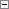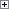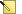The following functions perform various actions on fields:

• CALCFIELDS
• CALCSUMS
• FIELDERROR
• FIELDNAME
• INIT
• TESTFIELD
• VALIDATE

CALCFIELDS Function

CALCFIELDS updates FlowFields. FlowFields are automatically updated when they are the direct source expressions of controls, but they must be explicitly calculated when they are part of a more complex expression. For more information about flowfields, see FlowFields.

CALCFIELDS has the following syntax.Copy Code
[Ok :=] Record.CALCFIELDS(Field1, [Field2],...)

When you use FlowFields in C/AL functions, you must use the CALCFIELDS function to update them.

In the following example, the SETRANGE function sets a filter and then the CALCFIELDS function calculates the Balance and Balance Due fields by using the current filter and performing the calculations that are defined as the CalcFormula properties of the FlowFields. This example requires that you create the following variable.

Variable Data type Subtype

Customer

Record

CustomerCopy Code
Customer.GET('01454545');
Customer.SETRANGE("Date Filter",0D,TODAY);
Customer.CALCFIELDS(Balance,"Balance Due");
MESSAGE('The Balance is %1 and your Balance Due is %2',Customer.Balance,Customer."Balance Due");

The following message is displayed:

The Balance is 342,529.44 and your Balance Due is 342,529.44

CALCSUMS Function

CALCSUMS calculates the sum of one or more fields that are SumIndexFields in the record.

CALCSUMS has the following syntax.Copy Code
[Ok :=] Record.CALCSUMS (Field1, [Field2],...)

For CALCSUMS, a key that contains the SumIndexFields must be selected as the current key. Similar to CALCFIELDS, CALCSUMS uses the current filter settings when it performs the calculation.

In the following example, an appropriate key is selected, some filters are set, the calculation is performed and then a message is displayed. This example requires that you create the following variable.

Variable Data type Subtype

custledgerentry

Record

Cust. Ledger EntryCopy Code
custledgerentry.SETCURRENTKEY("Customer No.");
custledgerentry.SETRANGE("Customer No.",'10000','50000');
custledgerentry.SETRANGE("Posting Date",0D,TODAY);
custledgerentry.CALCSUMS("Sales (LCY)");
MESSAGE ('%1 calculated sales',custledgerentry."Sales (LCY)")

FIELDERROR Function

FIELDERROR triggers a run-time error after it displays a field-related error message.

FIELDERROR has the following syntax.Copy Code
Record.FIELDERROR(Field, [Text])

This function is very similar to the ERROR function. However, in the FIELDERROR function, if the name of a field is changed, for example, translated to another language, in the Table Designer, the message from the FIELDERROR function will reflect the current name of the field.

The following examples show how to use the FIELDERROR function. These examples require that you create the following variable.

Variable Date type Subtype

Item

Record

ItemCopy Code
Item.GET('70000');
IF Item.Class <> 'HARDWARE' THEN
Item.FIELDERROR(Class);

If item 70000 has a Class other than HARDWARE, then you receive the following error message:

Class must not be OTHER in Item No. ='70000'.

If the text or code field contains the empty string, then you receive the following error message:

You must specify Class in Item No.='70000'.

If the field is a numeric field and is empty, it is treated as if it contains the value 0 (zero), and then you receive the following error message:

Class must not be 0 in Item No.='70000'.

You can change the default text that is displayed in the error message. The following example shows how to use the FIELDERROR function and change the default text. This example requires that you create the following variable.

Variable Data type

Class

CodeCopy Code
IF Item.Class < '4711' THEN
Item.FIELDERROR(Class,'must be greater than 4711');

The following error message is displayed:

Class must be greater than 4711 in Item No.='70000'.

FIELDNAME

FIELDNAME returns the name of a field. It has the following syntax.Copy Code
Name := Record.FIELDNAME(Field)

You could just use the name of the field. However, using FIELDNAME lets you create messages that always contain the name of the field, even if the name of the field is changed.

This example shows how to use FIELDNAME together with FIELDERROR.Copy Code
FIELDERROR(
Quantity,'must not be less than ' + FIELDNAME("Quantity Shipped"));

INIT

INIT initializes a record. It has the following syntax.Copy Code
Name := Record.FIELDNAME(Field)

If a default value for a field has been defined by using the InitValue property, this value is used for the initialization. Otherwise, the default value of each data type is usedNote
INIT does not initialize the fields of the primary key.

TESTFIELD Function

TESTFIELD tests whether a field contains a specific value. It has the following syntax.Copy Code
Record.TESTFIELD(Field, [Value])

If the test fails, that is, if the field does not contain the specified value, an error message is displayed and a run-time error is triggered. This means that any changes that were made to the record are discarded. If the value that you test against is an empty string, the field must have a value other than blank or 0 (zero).

The following example tests the Language Code field for customer number 10000 in the Customer table and tests whether the Language Code is ZX. This example requires that you create the following variable.

Variable Data type Subtype

customer

Record

CustomerCopy Code
customer.GET('10000')
customer.TESTFIELD("Language Code",'ZX');

VALIDATE Function

VALIDATE calls the OnValidate trigger of a field. It has the following syntax.Copy Code
Record.VALIDATE(Field [, NewValue])

When you enter an account number in a ledger, code in a table trigger is executed to transfer the name of the account from the chart of accounts. If you enter an account number in a batch job, the code which transfers the name of the account is not automatically executed. The following example executes the appropriate field-level trigger code. This example requires that you create the following variable.

Variable Data type Subtype

GeneralLedgerEntry

Record

G/L EntryCopy Code
GeneralLedgerEntry.VALIDATE("G/L AccountNo", '100');

This corresponds to the following code.Copy Code
GeneralLedgerEntry."G/L AccountNo" := '100';
GeneralLedgerEntry.VALIDATE("G/L AccountNo");

The VALIDATE function is useful for centralizing processing, which makes your application easier to maintain.

For example, if the OnValidate trigger of the Total Amount field performs a calculation that uses values from three other fields as operands, the calculation must be performed again if the contents of any one of these fields changes. You should avoid entering the calculation formula in the OnValidate triggers of each field because this can create errors if the calculation formula has to be changed later and you have to update the code in all the triggers. Instead, you should enter the calculation formula in the OnValidate trigger of only one of the fields and call this trigger code from the OnValidate triggers of the other fields.